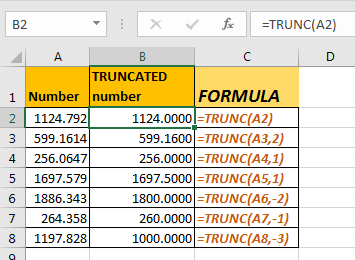# How to use the TRUNC function in Excel

The TRUNC function in Excel reduces a number to given number of digits. For example, using TRUNC function, you can make 125452.65 to 125452.00 or 125452.60 or 125000.00.
You may think that it is same as ROUND function in excel, but it is not. Instead of rounding the number to nearest 0 it just reduces the number to given 0’s. In English, as the name suggests it truncates the number to given digits.Syntax:

=TRUNC(number,[num_digits])

Number: the number you want to truncate.
[num_digits]: number of digits that you want to truncate. It's optional. By default, it is 0. If you omit this, all digits after the decimal point is reduced to 0.

Let’s take some quick examples of it.

Example 1

To truncate 125.551 to 1 digit. Write below formula.

=TRUNC(125.551,1)

This will return 125.500

Example 2

To truncate 125.501 to 2 digits, write below formula.

=TRUNC(125.551,2)

This will return 125.55.

Example 3

If you want to truncate all numbers after the decimal point, write below formula.

=TRUNC(125.551)

This will return 125.000.

Example 4

If you want to truncate a number to sum digits before decimal number, then use negative numbers. For example, if you wish to 12547 to be truncated as 12500 then write below formula.

=TRUNC(12547,-2)

So yeah guys, this how TRUNC formula is used in excel 2016. Let me know if you have any question regarding this or anything in excel in the comments section below.

Excel Round function

Excel RoundUp function

Excel Rounddown Function

Formula Round to nearest 100 and 1000

Rounding Numerical Calculation Results In Microsoft Excel

Popular Articles:

How to use the VLOOKUP Function in Excel

How to use the COUNTIF function in Excel 2016

How to Use SUMIF Function in Excel

1.Interesting

2.Interesting.

3.Depends on the reason for truncating numbers. For most part, I just edit the format of the text in the cell so I can show it in a truncated form while still being able to use it without issues of error propagation.

Terms and Conditions of use

The applications/code on this site are distributed as is and without warranties or liability. In no event shall the owner of the copyrights, or the authors of the applications/code be liable for any loss of profit, any problems or any damage resulting from the use or evaluation of the applications/code.